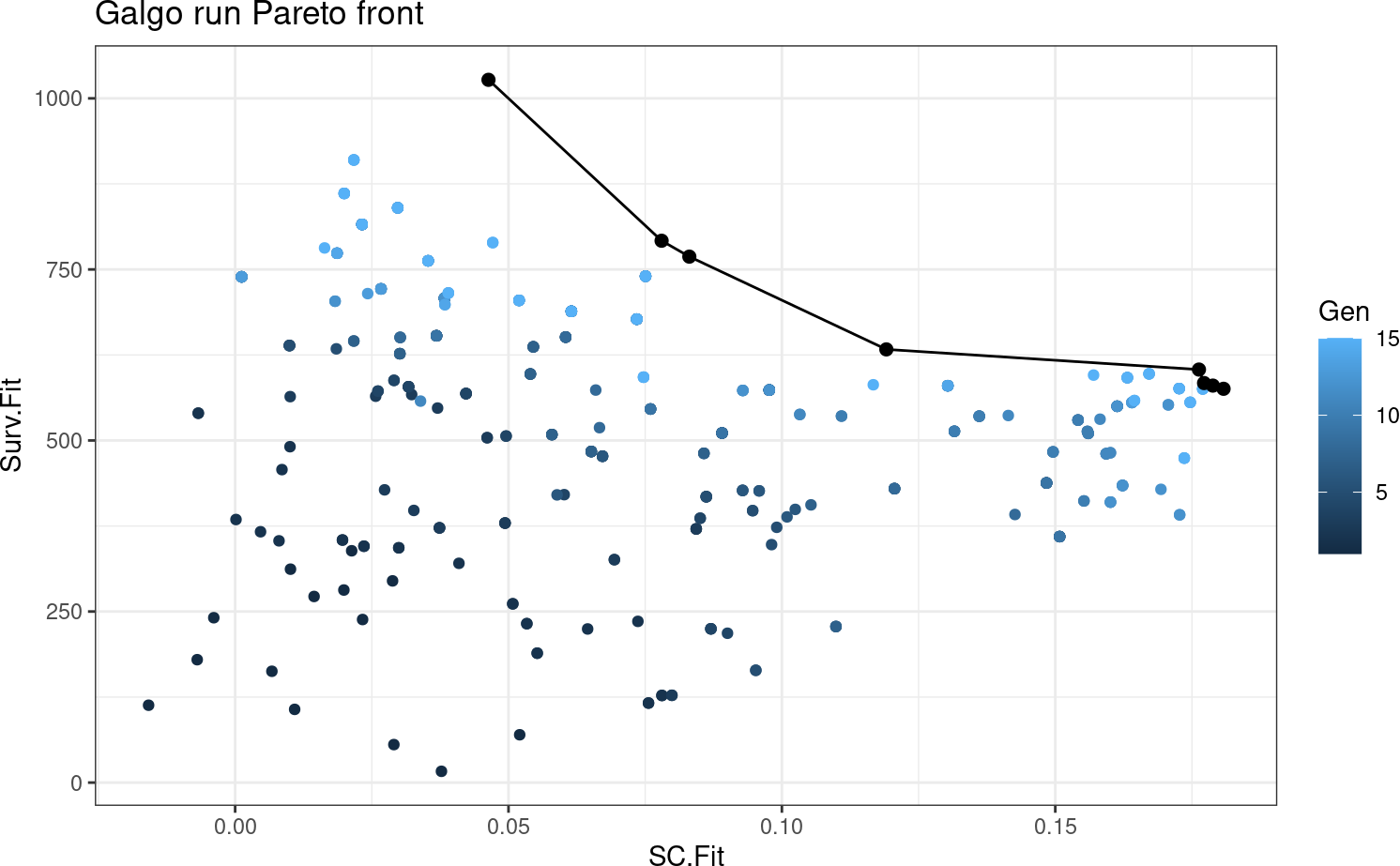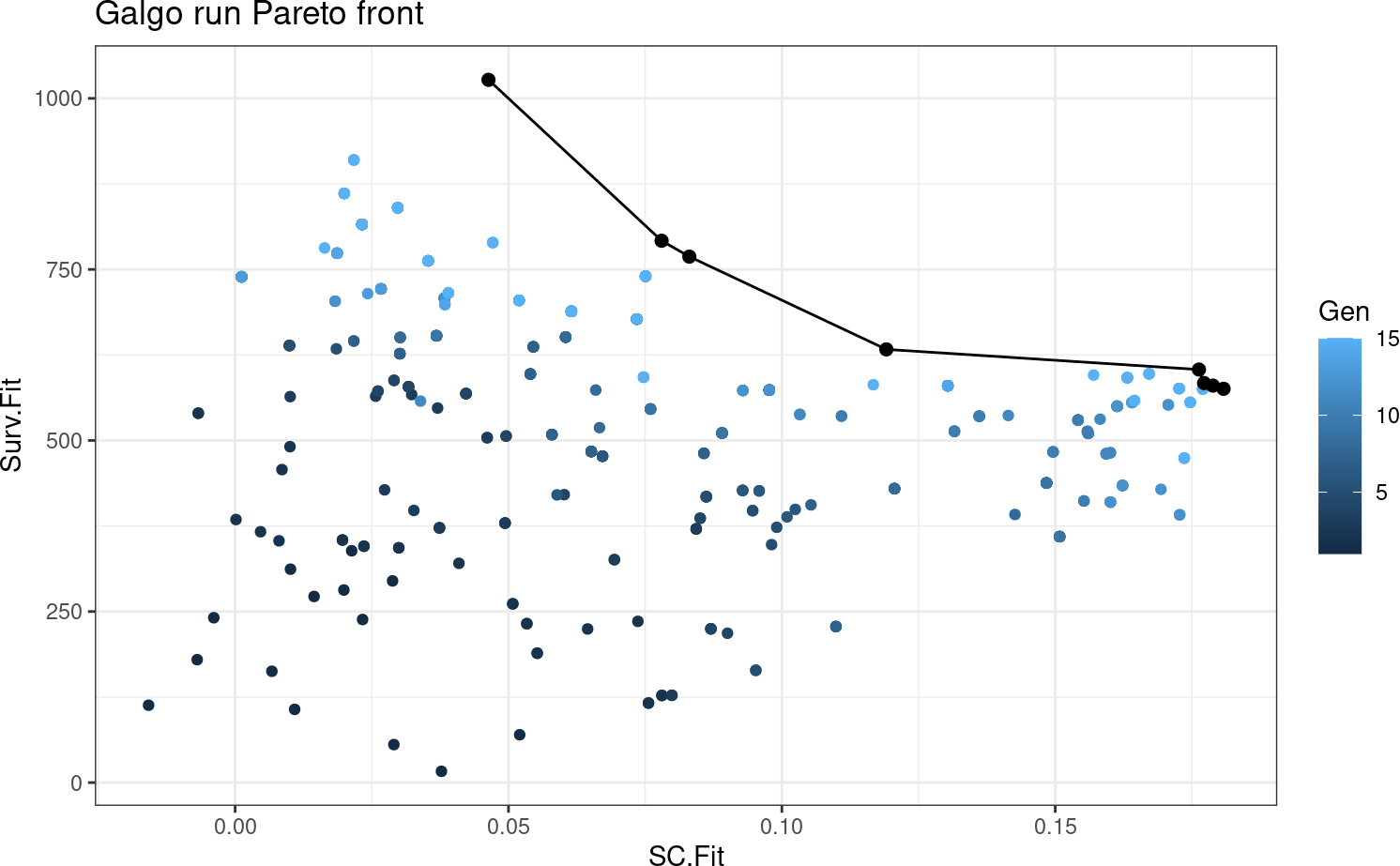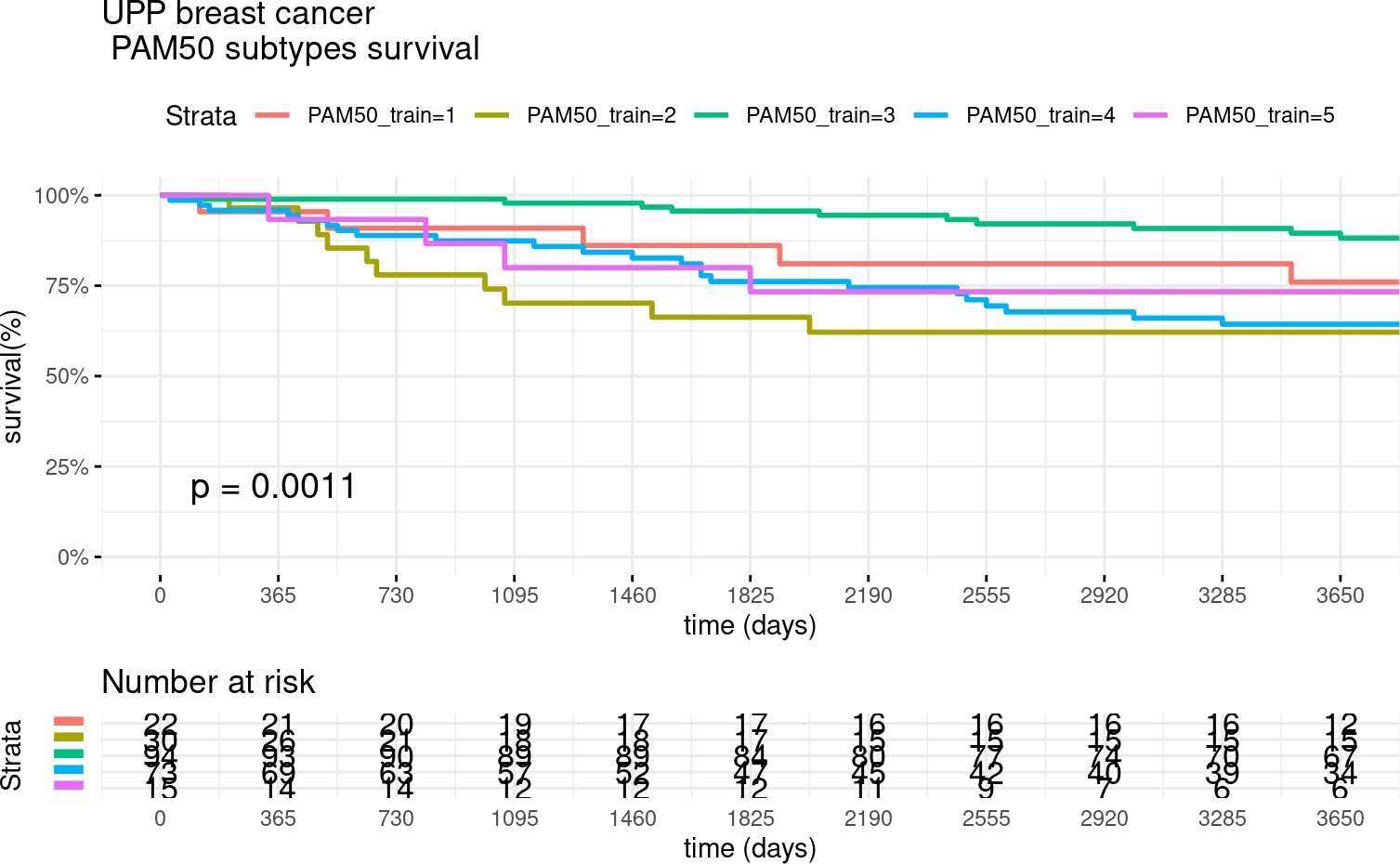# 1 Overview

In the new era of omics data, precision medicine has become the new paradigm of cancer treatment. Among all available omics techniques, gene expression profiling, in particular, has been increasingly used to classify tumor subtypes with different biological behavior. Cancer subtype discovery is usually approached from two possible perspectives:

-Using the molecular data alone with unsupervised techniques such as clustering analysis. -Using supervised techniques focusing entirely on survival data.

The problem of finding patients subgroups with survival differences while maintaining cluster consistency could be viewed as a bi-objective problem, where there is a trade-off between the separability of the different groups and the ability of a given signature to consistently distinguish patients with different clinical outcomes. This gives rise to a set of optimal solutions, also known as Pareto-optimal solutions. To overcome these issues, we combined the advantages of clustering methods for grouping heterogeneous omics data and the search properties of genetic algorithms in GSgalgoR: A flexible yet robust multi-objective meta-heuristic for disease subtype discovery based on an elitist non-dominated sorting genetic algorithm (NSGA-II), driven by the underlying premise of maximizing survival differences between groups while getting high consistency and robustness of the clusters obtained.

# 2 Algorithm

In the GSgalgoR package, the NSGA-II framework was used for finding multiple Pareto-optimal solutions to classify patients according to their gene expression patterns. Basically, NSGA-II starts with a population of competing individuals which are evaluated under a set of fitness functions that estimate the survival differences and cohesiveness of the different transcriptomic groups. Then, solutions are ranked and sorted according to their non-domination level which will affect the way they are chosen to be submitted to the so-called “evolutionary operators” such as crossover and mutation. Once a set of well-suited solutions are selected and reproduced, a new offspring of individuals composed of a mixture of the “genetic information” of the parents is obtained. Parents and offspring are pooled and the best-ranked solutions are selected and passed to the next generation which will start over the same process again.

# 3 Installation

## 3.1 GSgalgoR library

To install GSgalgoR package, start R and enter:

``````
if (!requireNamespace("BiocManager", quietly = TRUE))
install.packages("BiocManager")

BiocManager::install("GSgalgoR")
library(GSgalgoR)``````

Alternatively you can install GSgalgoR from github using the devtool package

``````devtools::install_github("https://github.com/harpomaxx/GSgalgoR")
library(GSgalgoR)``````

## 3.2 Examples datasets

To standardize the structure of genomic data, we use the ExpressionSet structure for the examples given in this guide. The `ExpressionSet` objects are formed mainly by:

• A matrix of genetic expression, usually derived from microarray or RNAseq experiments. - Phenotypic data, where we find information on the samples (condition, status, treatment, survival, and other covariates). - Finally, these objects can also contain Annotations and feature Meta-data.

To start testing GSgalgoR, we will use two Breast Cancer datasets. Namely, the UPP and the TRANSBIG datasets. Additionally, we will use PAM50 centroids to perform breast cancer sample classification. The datasets can be accessed from the following Bioconductor packages:

``````
BiocManager::install("breastCancerUPP",version = "devel")
BiocManager::install("breastCancerTRANSBIG",version = "devel")
``````
``````
library(breastCancerTRANSBIG)
library(breastCancerUPP)``````

Also, some basic packages are needed to run the example in this vignette

``````library(GSgalgoR)
library(Biobase)
library(genefu)
library(survival)
library(survminer)
library(ggplot2)
data(pam50)``````

# 4 Examples

To access the `ExpressionSets` we use:

``````data(upp)
Train<- upp
rm(upp)

data(transbig)
Test<- transbig
rm(transbig)

#To access gene expression data
train_expr<- exprs(Train)
test_expr<- exprs(Test)

#To access feature data
train_features<- fData(Train)
test_features<- fData(Test)

#To access clinical data
train_clinic <- pData(Train)
test_clinic <- pData(Test) ``````

## 4.2 Data tidying and preparation

Galgo can accept any numeric data, like probe intensity from microarray experiments or RNAseq normalized counts, nevertheless, features are expected to be scaled across the dataset before being plugged in into the Galgo Framework. For PAM50 classification, Gene Symbols are expected, so probesets are mapped into their respective gene symbols. Probesets mapping for multiple genes are expanded while Genes mapped to multiple probes are collapsed selecting the probes with the highest variance for each duplicated gene.

### 4.2.1 Drop duplicates and NA’s

``````
#Custom function to drop duplicated genes (keep genes with highest variance)

DropDuplicates<- function(eset, map= "Gene.symbol"){

#Drop NA's
drop <- which(is.na(fData(eset)[,map]))
eset <- eset[-drop,]

#Drop duplicates
drop <- NULL
Dup <- as.character(unique(fData(eset)[which(duplicated
(fData(eset)[,map])),map]))
Var <- apply(exprs(eset),1,var)
for(j in Dup){
pos <- which(fData(eset)[,map]==j)
drop <- c(drop,pos[-which.max(Var[pos])])
}

eset <- eset[-drop,]

featureNames(eset) <- fData(eset)[,map]
return(eset)
}``````

### 4.2.2 Expand probesets that map for multiple genes

``````
# Custom function to expand probesets mapping to multiple genes
expandProbesets <- function (eset, sep = "///", map="Gene.symbol"){
x <- lapply(featureNames(eset), function(x) strsplit(x, sep)[])
y<- lapply(as.character(fData(eset)[,map]), function(x) strsplit(x,sep))
eset <- eset[order(sapply(x, length)), ]
x <- lapply(featureNames(eset), function(x) strsplit(x, sep)[])
y<- lapply(as.character(fData(eset)[,map]), function(x) strsplit(x,sep))
idx <- unlist(sapply(1:length(x), function(i) rep(i,length(x[[i]]))))
idy <- unlist(sapply(1:length(y), function(i) rep(i,length(y[[i]]))))
xx <- !duplicated(unlist(x))
idx <- idx[xx]
idy <- idy[xx]
x <- unlist(x)[xx]
y <- unlist(y)[xx]
eset <- eset[idx, ]
featureNames(eset) <- x
fData(eset)[,map] <- x
fData(eset)\$gene <- y
return(eset)
}``````
``````Train=DropDuplicates(Train)
Train=expandProbesets(Train)
#Drop NAs in survival
Train <- Train[,!is.na(
survival::Surv(time=pData(Train)\$t.rfs,event=pData(Train)\$e.rfs))]

Test=DropDuplicates(Test)
Test=expandProbesets(Test)
#Drop NAs in survival
Test <-
Test[,!is.na(survival::Surv(
time=pData(Test)\$t.rfs,event=pData(Test)\$e.rfs))]

#Determine common probes (Genes)
Int= intersect(rownames(Train),rownames(Test))

Train= Train[Int,]
Test= Test[Int,]

identical(rownames(Train),rownames(Test))
#>  TRUE``````

For simplicity and speed, we will create a reduced expression matrix for the examples.

``````
#First we will get PAM50 centroids from genefu package

PAM50Centroids <- pam50\$centroids
PAM50Genes <- pam50\$centroids.map\$probe
PAM50Genes<- featureNames(Train)[ featureNames(Train) %in% PAM50Genes]

#Now we sample 200 random genes from expression matrix

Non_PAM50Genes<- featureNames(Train)[ !featureNames(Train) %in% PAM50Genes]
Non_PAM50Genes <- sample(Non_PAM50Genes,200, replace=FALSE)

reduced_set <- c(PAM50Genes, Non_PAM50Genes)

#Now we get the reduced training and test sets

Train<- Train[reduced_set,]
Test<- Test[reduced_set,]``````

### 4.2.3 Rescale expression matrix

Apply robust linear scaling as proposed in paper reference

``````
exprs(Train) <- t(apply(exprs(Train),1,genefu::rescale,na.rm=TRUE,q=0.05))
exprs(Test) <- t(apply(exprs(Test),1,genefu::rescale,na.rm=TRUE,q=0.05))

train_expr <- exprs(Train)
test_expr <- exprs(Test)``````

### 4.2.4 Survival Object

The ‘Surv’ object is created by the Surv() function of the survival package. This uses phenotypic data that are contained in the corresponding datasets, accessed by the `pData` command.

``````train_clinic <- pData(Train)
test_clinic <- pData(Test)

train_surv <- survival::Surv(time=train_clinic\$t.rfs,event=train_clinic\$e.rfs)
test_surv <- survival::Surv(time=test_clinic\$t.rfs,event=test_clinic\$e.rfs)``````

## 4.3 Run galgo()

The main function in this package is `galgo()`. It accepts an expression matrix and survival object to find robust gene expression signatures related to a given outcome. This function contains some parameters that can be modified, according to the characteristics of the analysis to be performed.

### 4.3.1 Setting parameters

The principal parameters are:

• population: a number indicating the number of solutions in the population of solutions that will be evolved
• generations: a number indicating the number of iterations of the galgo algorithm
• nCV: number of cross-validation sets
• distancetype: character, it can be ‘pearson’ (centered pearson), ‘uncentered’ (uncentered pearson), ‘spearman’ or ‘euclidean’
• TournamentSize: a number indicating the size of the tournaments for the selection procedure
• period: a number indicating the outcome period to evaluate the RMST
``````# For testing reasons it is set to a low number but ideally should be above 100
population <- 30
# For testing reasons it is set to a low number but ideally should be above 150
generations <-15
nCV <- 5
distancetype <- "pearson"
TournamentSize <- 2
period <- 3650``````

### 4.3.2 Run Galgo algorithm

``````set.seed(264)
output <- GSgalgoR::galgo(generations = generations,
population = population,
prob_matrix = train_expr,
OS = train_surv,
nCV = nCV,
distancetype = distancetype,
TournamentSize = TournamentSize,
period = period)``````
``````print(class(output))
#>  "galgo.Obj"
#> attr(,"package")
#>  "GSgalgoR"``````

### 4.3.3 Galgo Object

The output of the galgo() function is an object of type `galgo.Obj` that has two slots with the elements:

• Solutions
• ParetoFront.

#### 4.3.3.1 Solutions

Is a l x (n + 5) matrix where n is the number of features evaluated and l is the number of solutions obtained.

• The submatrix l x n is a binary matrix where each row represents the chromosome of an evolved solution from the solution population, where each feature can be present (1) or absent (0) in the solution.
• Column n+1 represent the k number of clusters for each solutions
• Column n+2 shows the SC Fitness
• Column n+3 represent Survival Fitness values
• Column n+4 shows the solution rank
• Column n+5 represent the crowding distance of the solution in the final pareto front

#### 4.3.3.2 ParetoFront

Is a list of length equal to the number of generations run in the algorithm. Each element is a l x 2 matrix where l is the number of solutions obtained and the columns are the SC Fitness and the Survival Fitness values respectively.

For easier interpretation of the `galgo.Obj`, the output can be transformed to a `list` or to a `data.frame` objects.

## 4.4 to_list() function

This function restructurates a `galgo.Obj` to a more easy to understand an use list. This output is particularly useful if one wants to select a given solution and use its outputs in a new classifier. The output of type list has a length equals to the number of solutions obtained by the galgo algorithm.

Basically this output is a list of lists, where each element of the output is named after the solution’s name (solution.n, where n is the number assigned to that solution), and inside of it, it has all the constituents for that given solution with the following structure:

• solution.n\$Genes: A vector of the features included in the solution
• solution.n\$k: The number of partitions found in that solution
• solution.n\$SC.Fit: The average silhouette coefficient of the partitions found
• solution.n\$Surv.Fit: The survival fitnes value
• solution.n\$Rank: The solution rank
• CrowD: The solution crowding distance related to the rest of the solutions
``````outputList <- to_list(output)
#>  "Solution.1" "Solution.2" "Solution.3" "Solution.4" "Solution.5"
#>  "Solution.6"``````

To evaluate the structure of the first solution we can run:

``````outputList[["Solution.1"]]
#> \$Genes
#>   "PHGDH"     "MYBL2"     "RRM2"      "SFRP1"     "BIRC5"     "UBE2C"
#>   "CDH3"      "PTTG1"     "BCL2"      "MMP11"     "CDC6"      "MELK"
#>  "MAPT"      "KRT14"     "KIF2C"     "FGFR4"     "MKI67"     "TYMS"
#>  "MLPH"      "CEP55"     "ACTR3B"    "CCNB1"     "MDM2"      "APCS"
#>  "TCTN2"     "ECE1"      "ORAI3"     "LOC441601" "SEMA3G"    "PHLDA3"
#>  "GRIK5"     "TMEM92"    "RFPL1"     "SERPINA3"  "DOK2"      "THSD4"
#>  "SGCD"      "GLYAT"     "PDE6B"     "C12orf39"  "EEF1A1P9"  "RABGEF1"
#>  "NIPBL"     "TARP"      "CASC1"     "GPC1"      "RAP1BL"    "LOC641298"
#>  "IFT20"     "MTCP1"     "LY6G5B"    "MORC3"     "GUCY2D"    "AGPAT2"
#>  "HBS1L"     "ACOT13"    "PTRH2"     "PLA2G2D"   "TRAF6"     "SEMA4C"
#>  "CBLN1"     "HTRA1"     "GSTO1"     "PSME4"     "BAIAP2"    "MAPK7"
#>  "TRBV7-7"   "MAP4K4"    "DAAM1"     "WDR41"     "SIN3B"     "WDHD1"
#>  "CNBP"      "HERC2P3"   "MAVS"      "FAM184A"   "CASP7"     "STMN2"
#>  "NEK4"      "SLC12A5"   "ARFGAP3"   "KIAA1539"  "MLL"       "ITGB3"
#>  "TAF1C"     "SUPV3L1"   "CHMP7"     "CDO1"      "UNC13B"    "RPS4XP9"
#>  "EPS8"      "CDK20"     "PCDH8"     "FGFR1"     "NFYB"      "DAAM2"
#>  "RALYL"     "RAB3GAP1"
#>
#> \$k
#>  2
#>
#> \$SC.Fit
#>  0.1807798
#>
#> \$Surv.Fit
#>  575.5382
#>
#> \$rank
#>  1
#>
#> \$CrowD
#>  Inf``````

## 4.5 to_dataframe() function

The current function restructures a `galgo.Obj` to a more easy to understand an use `data.frame`. The output data frame has m x n dimensions, were the rownames (m) are the solutions obtained by the galgo algorithm. The columns has the following structure:

• Genes: The features included in each solution in form of a list
• k: The number of partitions found in that solution
• SC.Fit: The average silhouette coefficient of the partitions found
• Surv.Fit: The survival fitness value
• Rank: The solution rank
• CrowD: The solution crowding distance related to the rest of the solutions
``````outputDF <- to_dataframe(output)
#>                    Genes k     SC.Fit  Surv.Fit Rank     CrowD
#> Solutions.1 PHGDH, M.... 2 0.18077977  575.5382    1       Inf
#> Solutions.2 PHGDH, E.... 6 0.04633810 1027.0871    1       Inf
#> Solutions.3 PHGDH, R.... 2 0.11910264  633.1157    1 0.6787695
#> Solutions.4 PHGDH, R.... 4 0.07799913  791.9089    1 0.4591678
#> Solutions.5 PHGDH, M.... 4 0.08307035  768.6752    1 0.3845864
#> Solutions.6 MYBL2, E.... 2 0.17627943  603.6453    1 0.3699591``````

## 4.6 plot_pareto()

Once we obtain the `galgo.obj` from the output of `galgo()` we can plot the obtained Pareto front and see how it evolved trough the tested number of generations

``plot_pareto(output)``# 5 Case study

Breast cancer (BRCA) is the most common neoplasm in women to date and one of the best studied cnacer types. Currently, numerous molecular alteration for this type of cancer are well known and many transcriptomic signatures have been developed for this type of cancer. In this regards, Perou et al. proposed one of the first molecular subtype classification according to transcriptomic profiles of the tumor, which recapitulates naturally-occurring gene expression patterns that encompass different functional pathways and patient outcomes. These subtypes, (LumA, LumB, Basal-like, HER2 and Normal-Like) have a strong overlap with the classical histopathological classification of BRCA tumors and might affect decision making when used to decided chemotherapy in certain cases.

## 5.1 Data Preprocessing

To evaluate Galgo’s performance along with PAM50 classification, we will use the two already scaled and reduced BRCA gene expression datasets and will compare Galgo performance with the widely used intrinsic molecular subtype PAM50 classification. Galgo performs feature selection by design, so this step is not strictly necessary to use galgoR (although feature selection might fasten GSgalgoRruns), nevertheless, appropriate gene expression scaling is critical when running GSgalgoR.

## 5.2 Breast cancer classification

The scaled expression values of each patient are compared with the prototypical centroids using Pearson’s correlation coefficient and the closest centroid to each patient is used to assign the corresponding labels.

``````#The reduced UPP dataset will be used as training set
train_expression <- exprs(Train)
train_clinic<- pData(Train)
train_features<- fData(Train)
train_surv<- survival::Surv(time=train_clinic\$t.rfs,event=train_clinic\$e.rfs)

#The reduced TRANSBIG dataset will be used as test set

test_expression <- exprs(Test)
test_clinic<- pData(Test)
test_features<- fData(Test)
test_surv<- survival::Surv(time=test_clinic\$t.rfs,event=test_clinic\$e.rfs)

#PAM50 centroids
centroids<- pam50\$centroids
#Extract features from both data.frames
inBoth<- Reduce(intersect, list(rownames(train_expression),rownames(centroids)))

#Classify samples

PAM50_train<- cluster_classify(train_expression[inBoth,],centroids[inBoth,],
method = "spearman")
table(PAM50_train)
#> PAM50_train
#>  1  2  3  4  5
#> 22 30 94 73 15

PAM50_test<- cluster_classify(test_expression[inBoth,],centroids[inBoth,],
method = "spearman")
table(PAM50_test)
#> PAM50_test
#>  1  2  3  4  5
#> 45 26 80 44  3

# Classify samples using genefu
#annot<- fData(Train)
#colnames(annot)="Gene.Symbol"
#PAM50_train<- molecular.subtyping(sbt.model = "pam50",
#         data = t(train_expression), annot = annot,do.mapping = TRUE)``````

Once the patients are classified according to their closest centroids, we can now evaluate the survival curves for the different types in each of the datasets

### 5.2.1 Survival of UPP patients

``````surv_formula <-
as.formula("Surv(train_clinic\$t.rfs,train_clinic\$e.rfs)~ PAM50_train")
tumortotal1 <- surv_fit(surv_formula,data=train_clinic)
tumortotal1diff <- survdiff(surv_formula)
tumortotal1pval<- pchisq(tumortotal1diff\$chisq, length(tumortotal1diff\$n) - 1,
lower.tail = FALSE)

p<-ggsurvplot(tumortotal1,
data=train_clinic,
risk.table=TRUE,
pval=TRUE,
palette="dark2",
title="UPP breast cancer \n PAM50 subtypes survival",
surv.scale="percent",
conf.int=FALSE,
xlab="time (days)",
ylab="survival(%)",
xlim=c(0,3650),
break.time.by = 365,
ggtheme = theme_minimal(),
risk.table.y.text.col = TRUE,
risk.table.y.text = FALSE,censor=FALSE)
print(p)``````### 5.2.2 Survival of TRANSBIG patients

``````surv_formula <-
as.formula("Surv(test_clinic\$t.rfs,test_clinic\$e.rfs)~ PAM50_test")
tumortotal2 <- surv_fit(surv_formula,data=test_clinic)
tumortotal2diff <- survdiff(surv_formula)
tumortotal2pval<- pchisq(tumortotal2diff\$chisq, length(tumortotal2diff\$n) - 1,
lower.tail = FALSE)

p<-ggsurvplot(tumortotal2,
data=test_clinic,
risk.table=TRUE,
pval=TRUE,
palette="dark2",
title="TRANSBIG breast cancer \n PAM50 subtypes survival",
surv.scale="percent",
conf.int=FALSE,
xlab="time (days)",
ylab="survival(%)",
xlim=c(0,3650),
break.time.by = 365,
ggtheme = theme_minimal(),
risk.table.y.text.col = TRUE,
risk.table.y.text = FALSE,
censor=FALSE)
print(p)``````